March 16, 2018

## The dictionary says

cumulative | ˈkyo͞omyələdiv, ˈkyo͞omyəˌlādiv |
§ increasing, accumulative, growing, mounting; collective, aggregate, amassed.
§ the cumulative effect of two years of drought
§ the effects of pollution are cumulative

## Cumulative sum

Everyday you spend some money

How much money you have each day?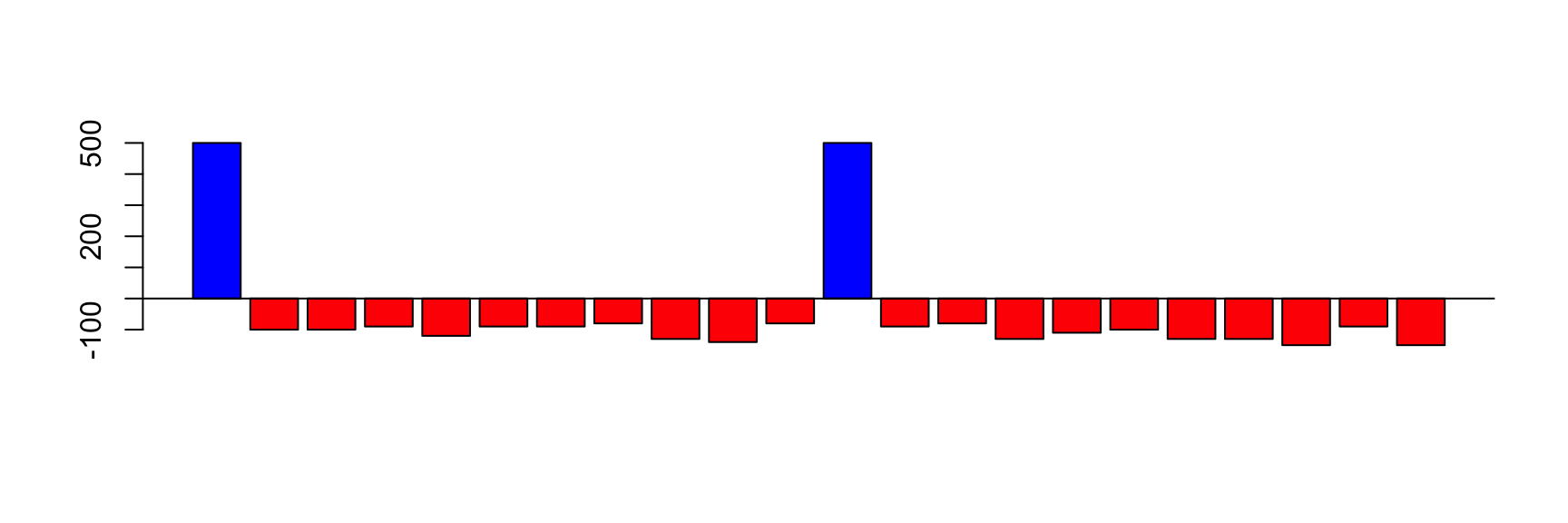## Cumulative Sum: daily balance

The income can be positive (salary) or negative (expenses)

The balance on first day is your initial money

The balance of today is the balance of yesterday plus the income of today

$\text{balance}[today]=\text{balance}[yesterday] + \text{income}[today]$

## balance and income are vectors

Since they have different values every day, we represent the income on day i by income[i]

We also represent the balance on day i by balance[i]

The vector income has the values for all days

The element income[i] has the value for a single day

## If we know income, we can calculate balance

The balance on first day is your initial money

balance <- income

The balance of today is the balance of yesterday plus the income of today

for(i in 2:length(income)) {
balance[i] <- balance[i-1] + income[i]
}

## Balance looks like this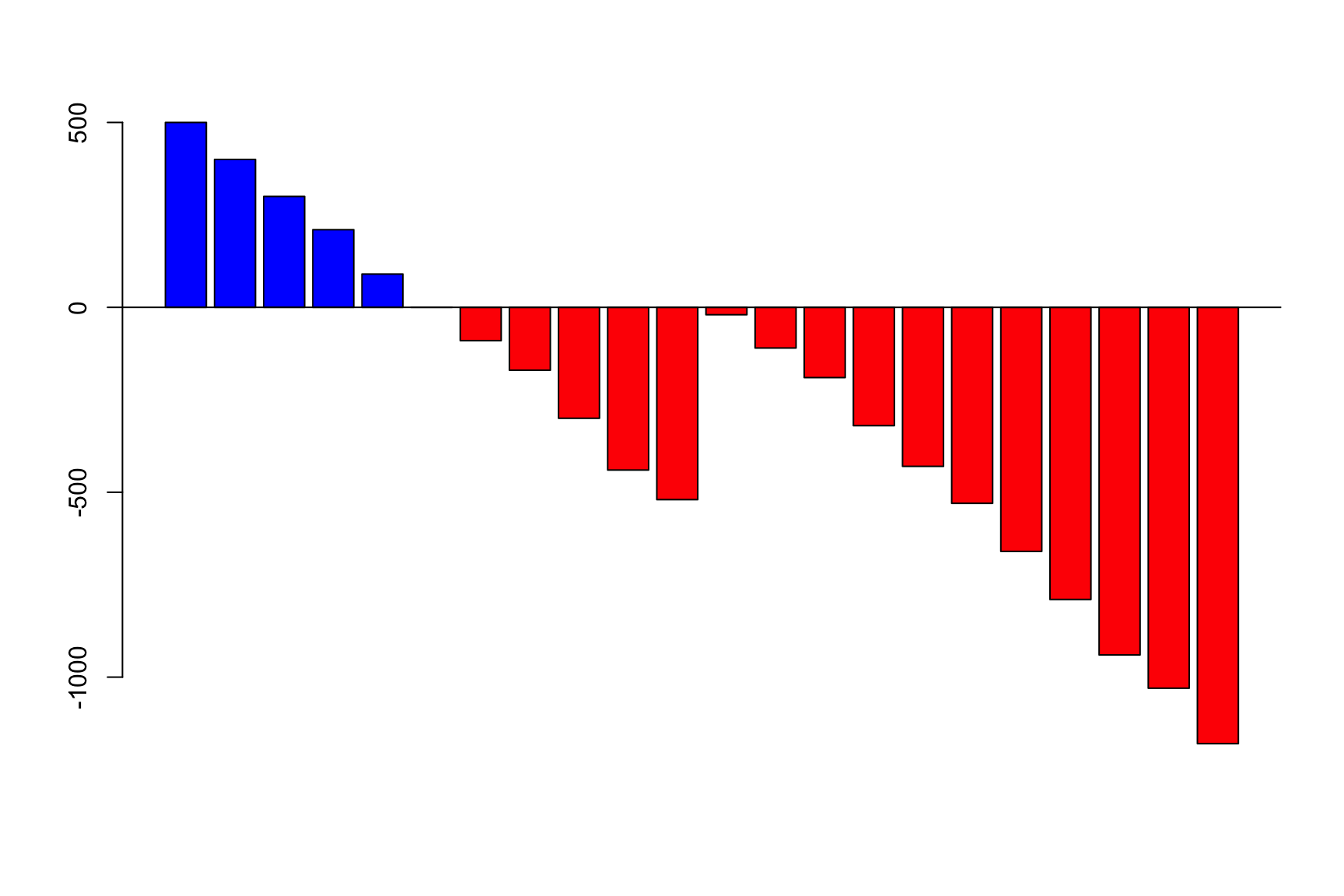## The same idea applies to GC-skew

The GC skew in each window is like the income in each day

And we want to see the “balance”

cum_skew <- skew
for(i in 2:length(skew)) {
cum_skew[i] <- cum_skew[i-1] + skew[i]
}

Same pattern, same solution

## Let’s see it with Carsonella ruddii

library(seqinr)
dna <- sequences[]

This bacteria has a very small genome, near 150 Kbp

So it is easy to use in classes

## Skew and Cum_skew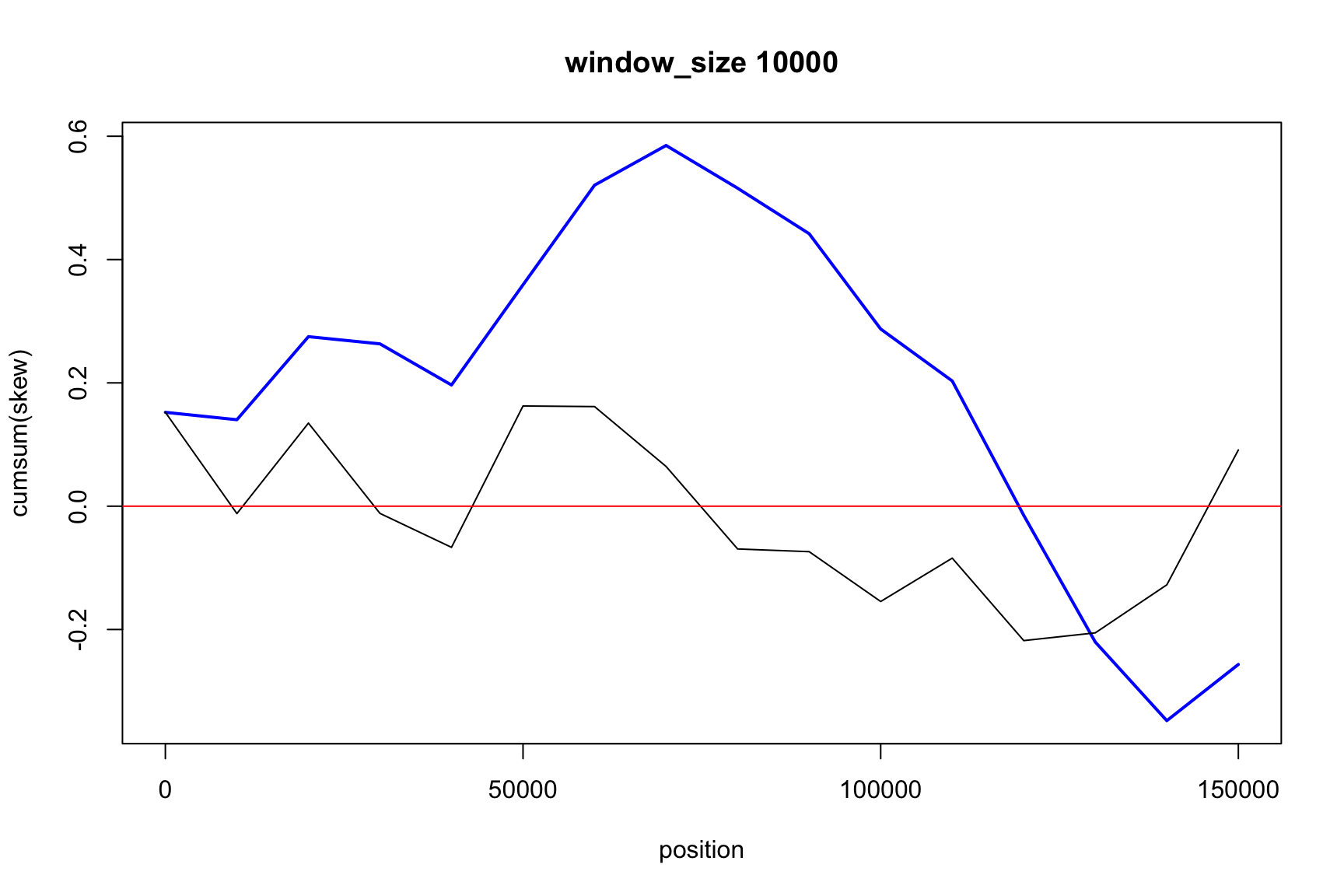## Skew and Cum_skew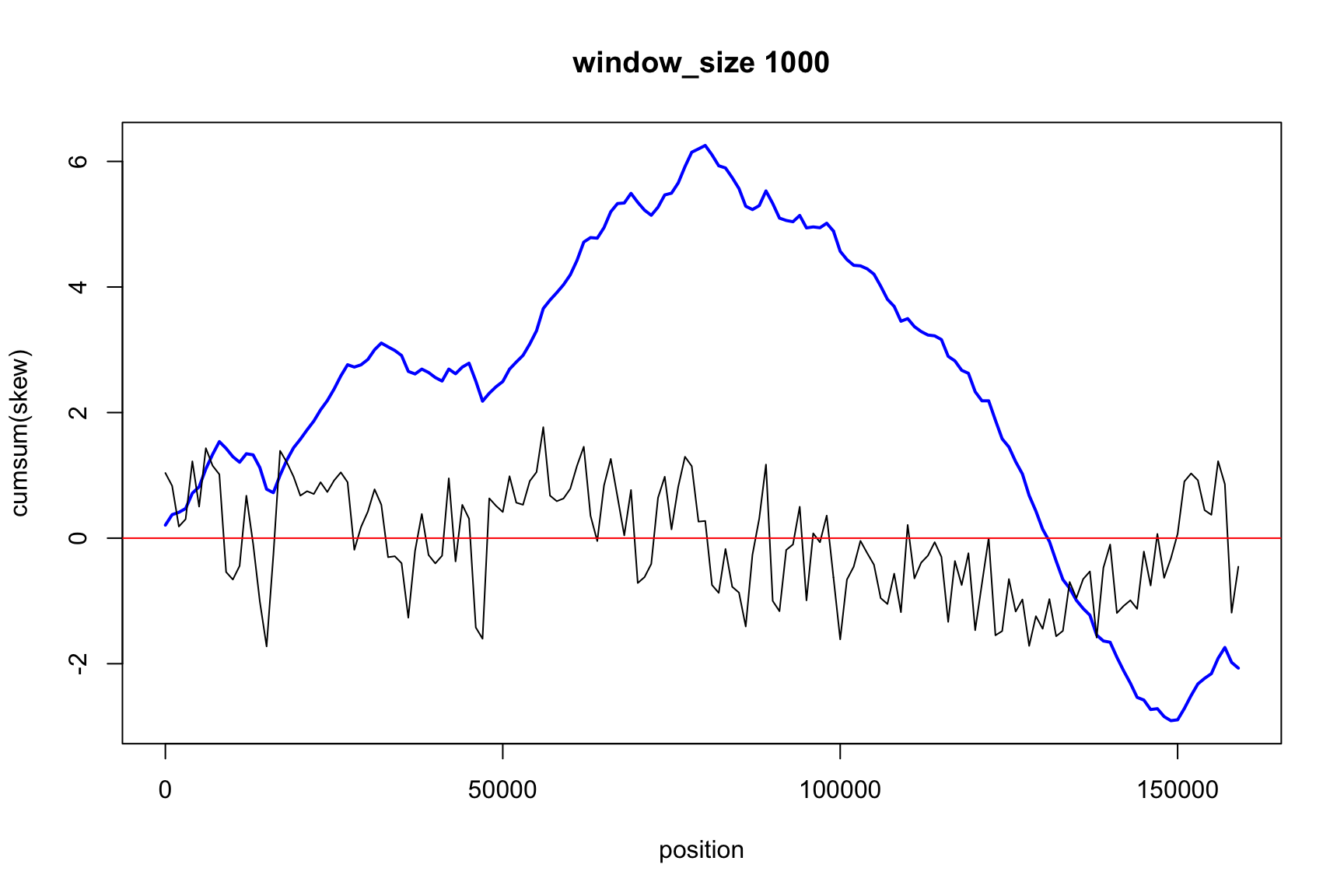## Skew and Cum_skew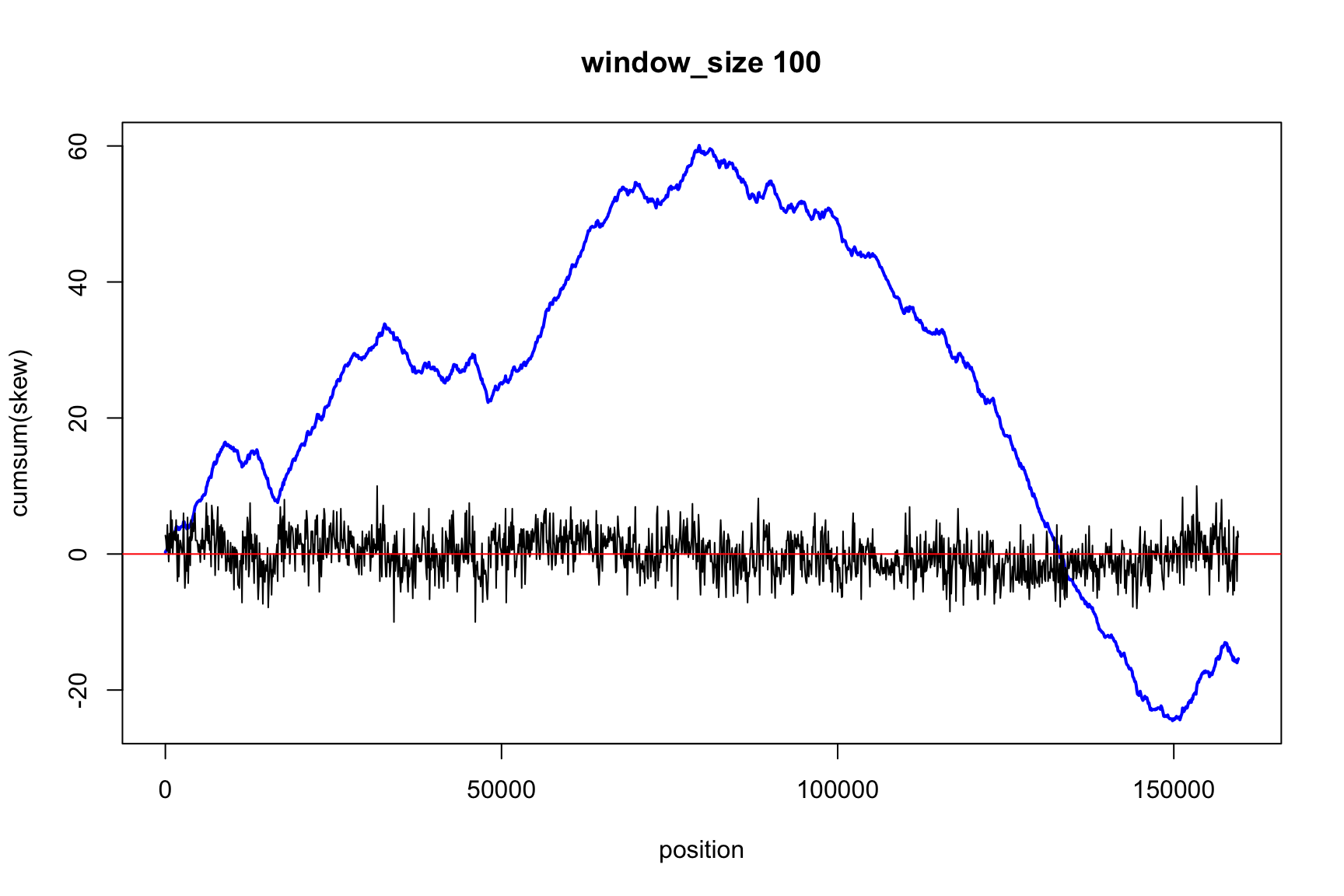## Skew and Cum_skew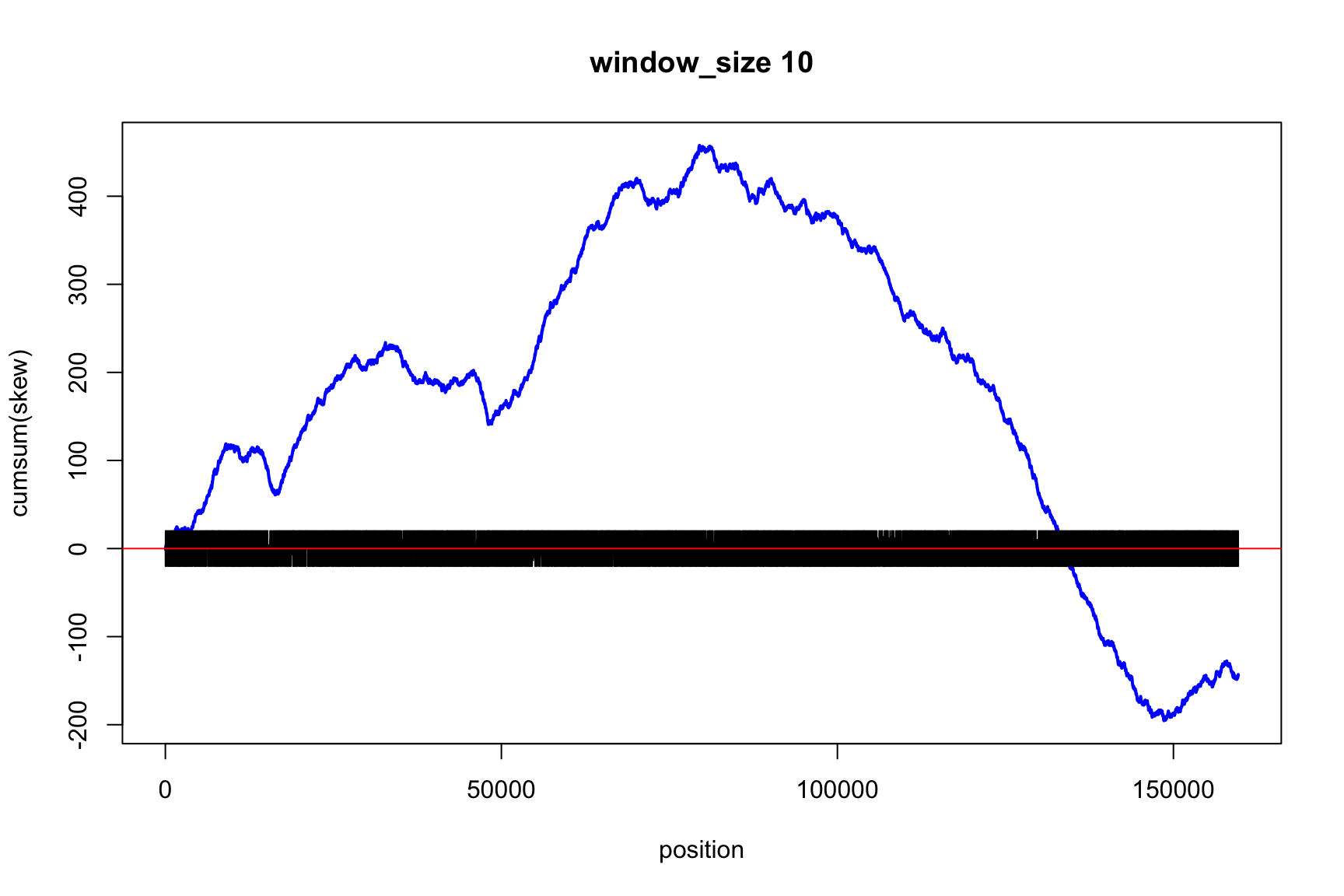## How small can window_size be?

We can make the window very small

If window_size=1,
How would be skew and cum_skew?

## One window = one nucleotide

If window_size=1 then we don’t need windows

Instead we use a simple rule

• If dna[i]=="g" then skew[i] <- 1
• If dna[i]=="c" then skew[i] <- -1
• If dna[i]=="a" or dna[i]=="t" then skew[i] <-0

How you do that in R?

## skew changes fast, cum_skew changes slow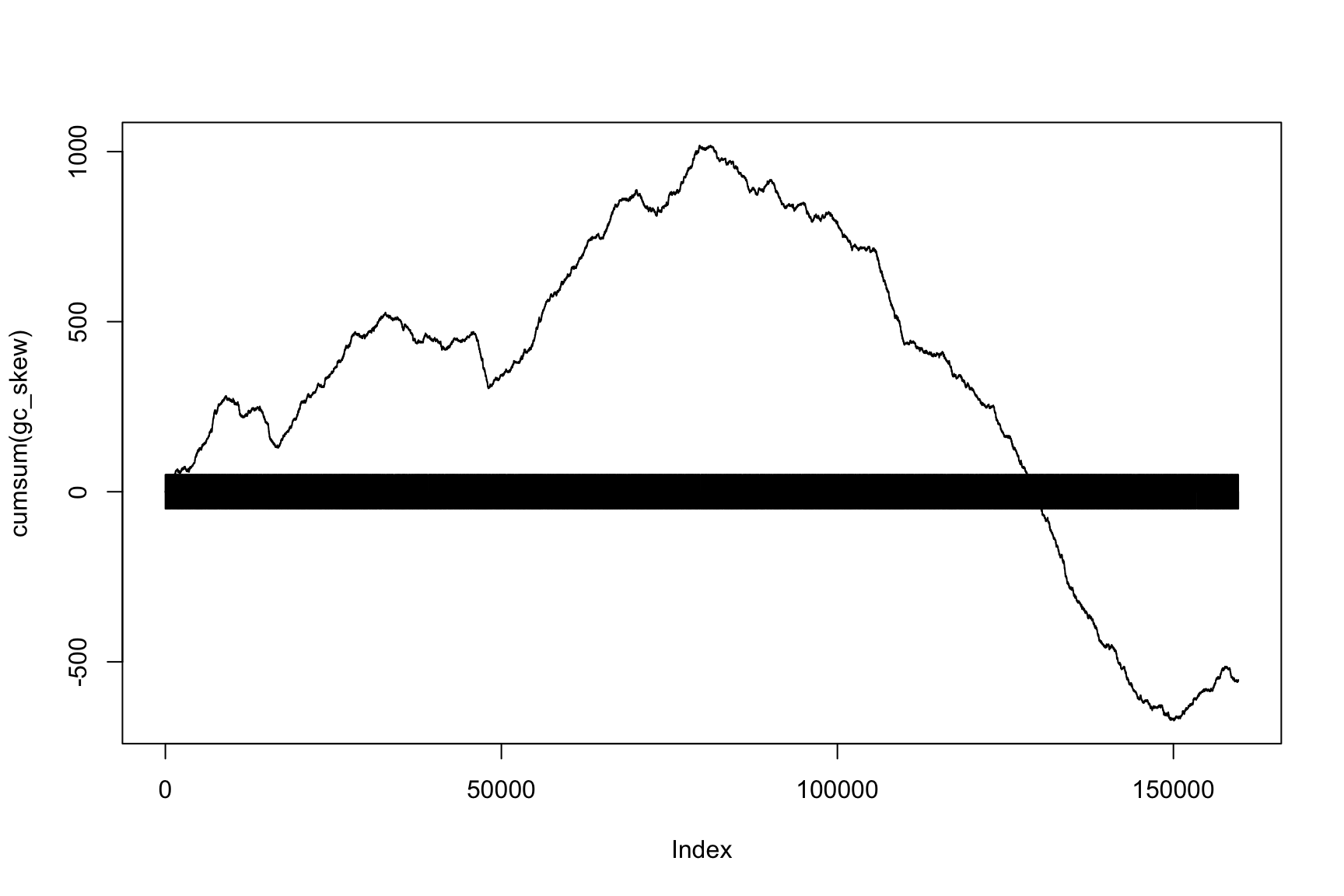## Let’s do the same with AT-skew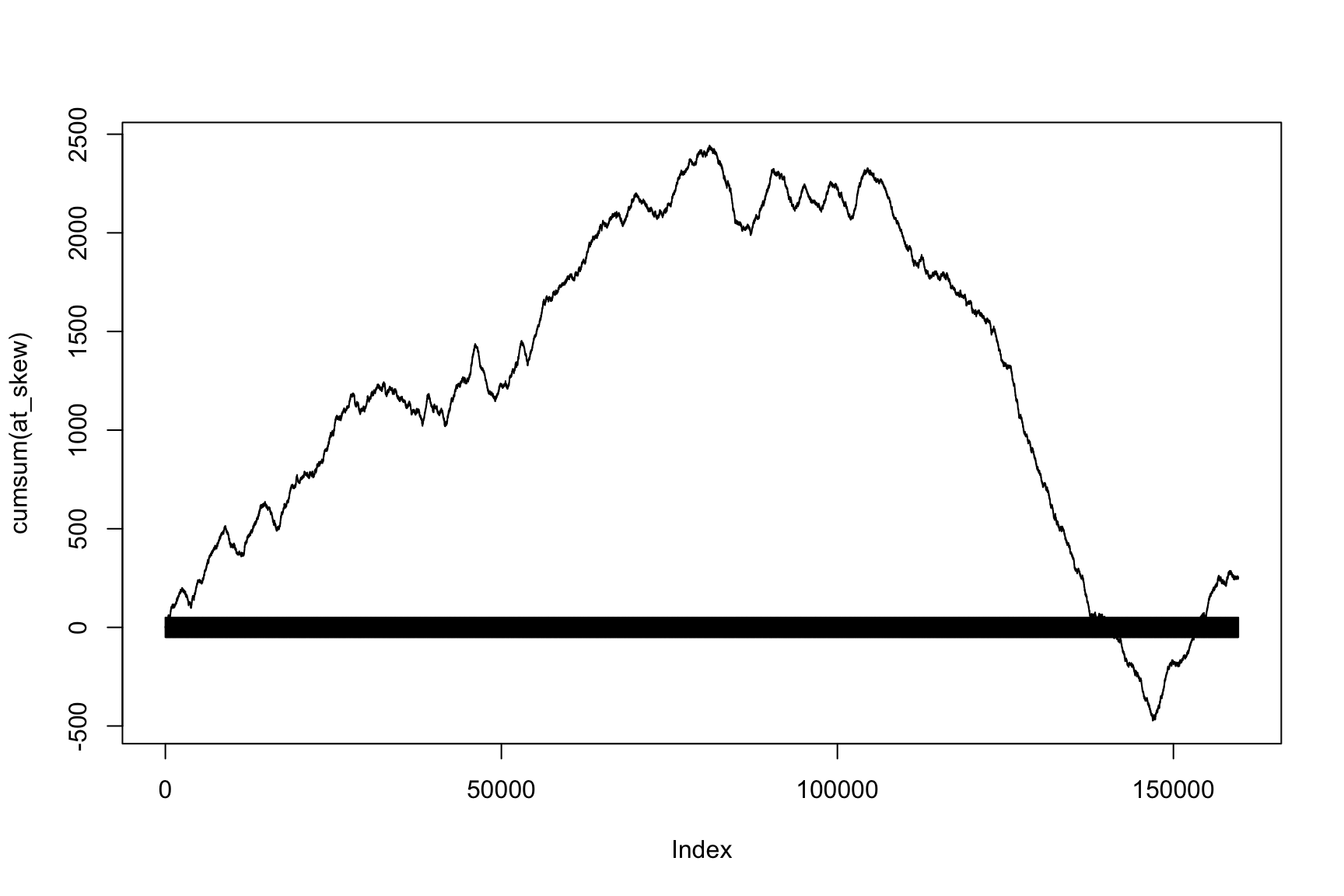## Last two plots depend on position

First plot is GC-skew versus Position

Second plot is AT-skew versus Position

What is the relationship between GC-skew and AT-skew?

How can you show it?

## GC-skew v/s AT-Skew

### Have we seen this before?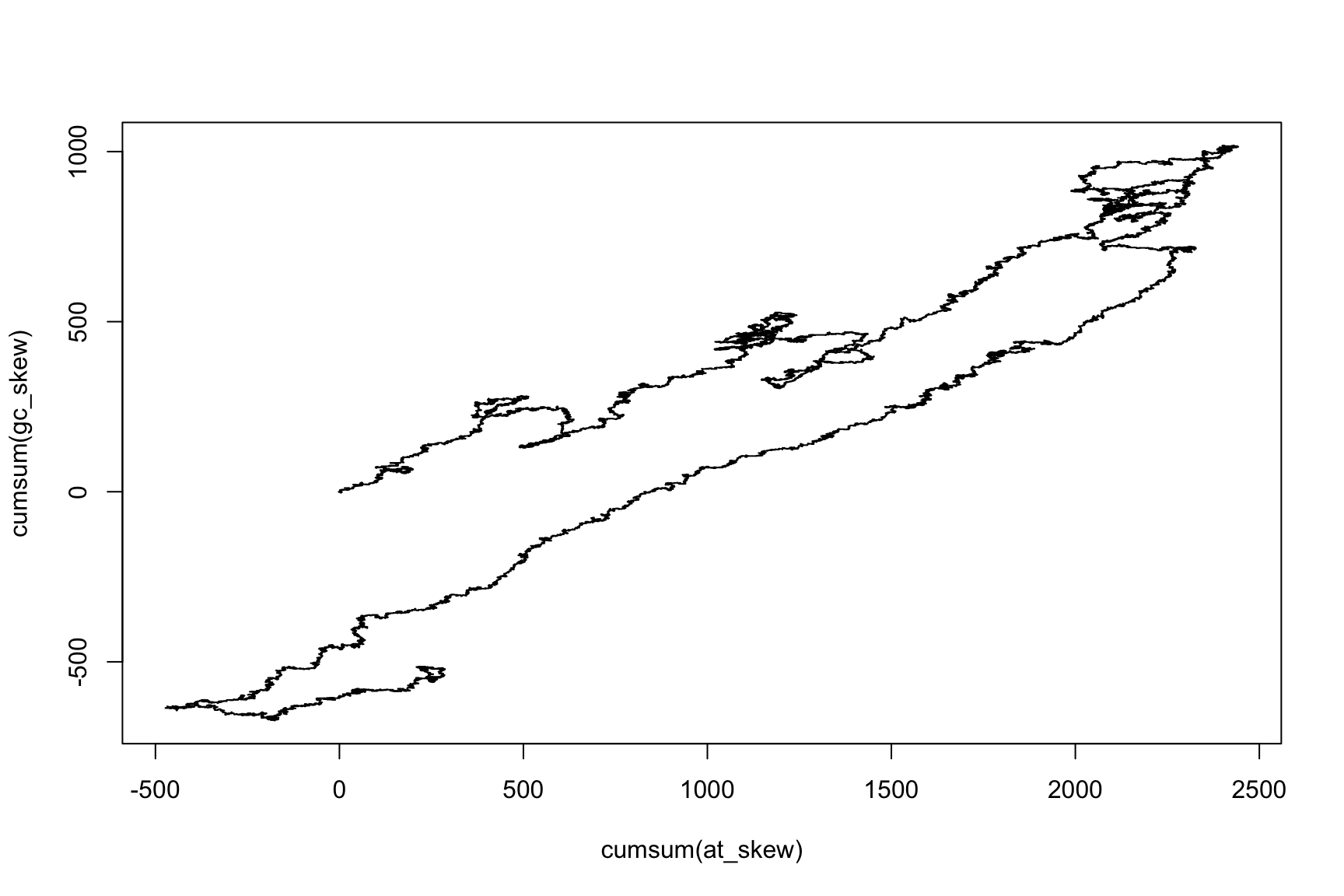## What else can we do?

• If we know the income, we can get balance
• If we know the skew, we can get cum_skew
• If we know the change, we can know the state

## Basic Systems Theory

These ideas are useful to understand the reality

A System is a set of parts that interact

Each part can be a smaller system

The behavior of the system depends on the parts AND on the interactions

## Total is bigger than the sum of the parts

Complex systems can have emergent properties

That is, the system can do things that the parts cannot

The system is different than each of the parts

## A simple system: growing cell lines

In a Petri dish we have two cells

Every hour, half of the cells replicate, and they never die

How many cells we have after i hours?

## The parts of the system

There is only one element: the cells

There are two values important to them:

• The number of cells
• The change in the number of cells

## Let’s give them names

The vector ncells is the number of cells

The value ncells[i] is the number of cells on time i

The vector growth is the growth of the number of cells

There are growth[i] new cells between time i-1 and i

## Solving the system

Let’s simulate for 100 hours

First we create empty vectors to store the result

ncells <- rep(NA, 100)
growth <- rep(NA, 100)

ncells <- 2
growth <- 0

## Every hour, half of the cells replicate

growth[i] <- ncells[i-1]/2

The growth on time i depends on the cells existing on time i-1

The income of today depends on the balance of yesterday

## The number of cells increases

ncells will be the cumulative sum of growth

But growth depends on ncells

Therefore, we have to calculate both together

## Then, time goes by

How many cells we have after 100 hours?

for(i in 2:100) {
growth[i] <- ncells[i-1]/2
ncells[i] <- ncells[i-1] + growth[i]
}
ncells
 5.420816e+17

## Plot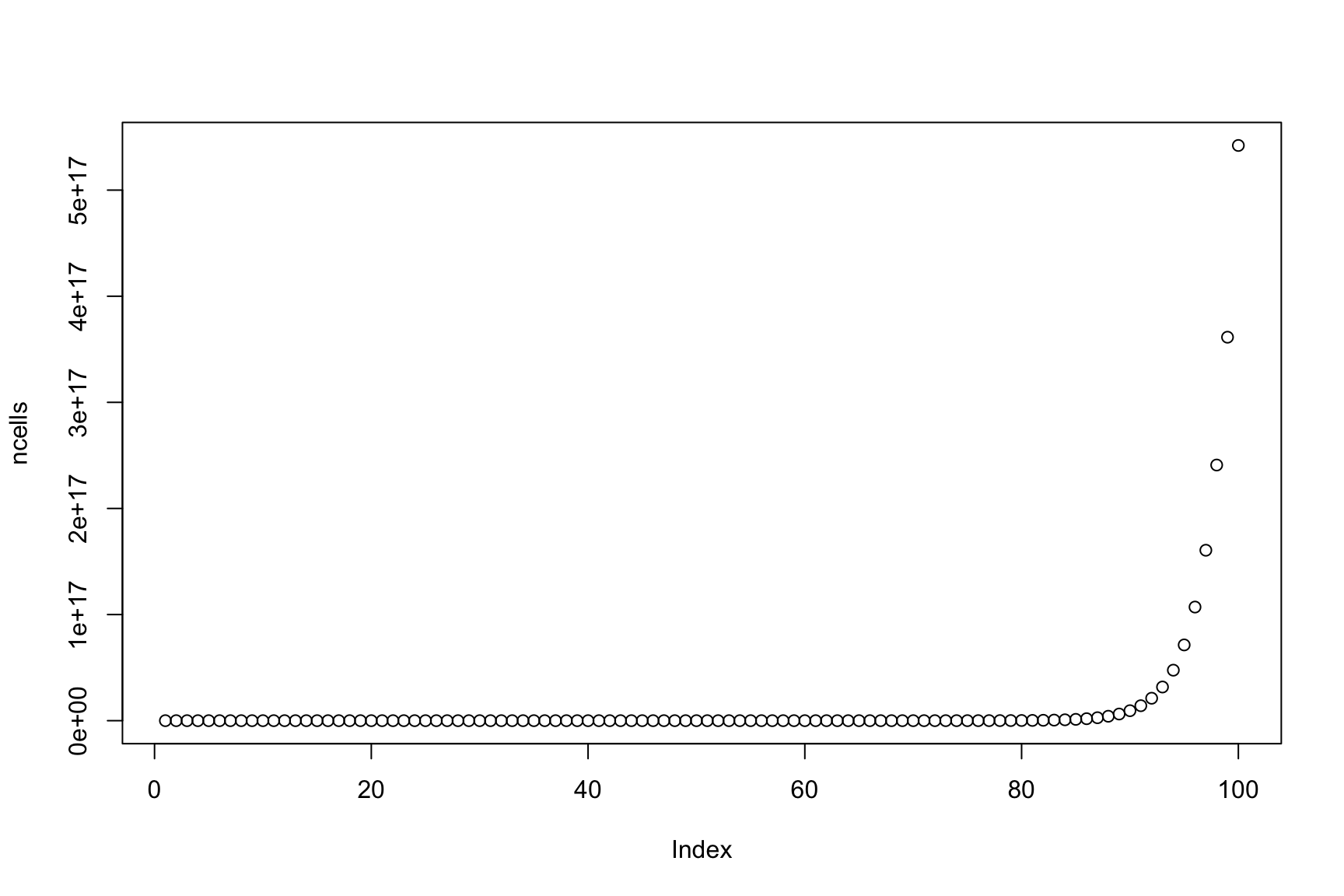## Limited food

In practice cells never grow that much

There is a limited amount of food

So the system has a second part: food

We represent it with the vector food

## Cells eat food, food limits growth rate

Let’s say that initially food <- 1E6

Each cell eats 1 food every hour: eaten[i] <- ncells[i-1]

Now growth depends on food:
growth[i] <- ncells[i-1]*food[i-1]/1E6

What happens now?

## Exercise

Make a program to simulate this system

What happens when food finish?

Is that realistic?